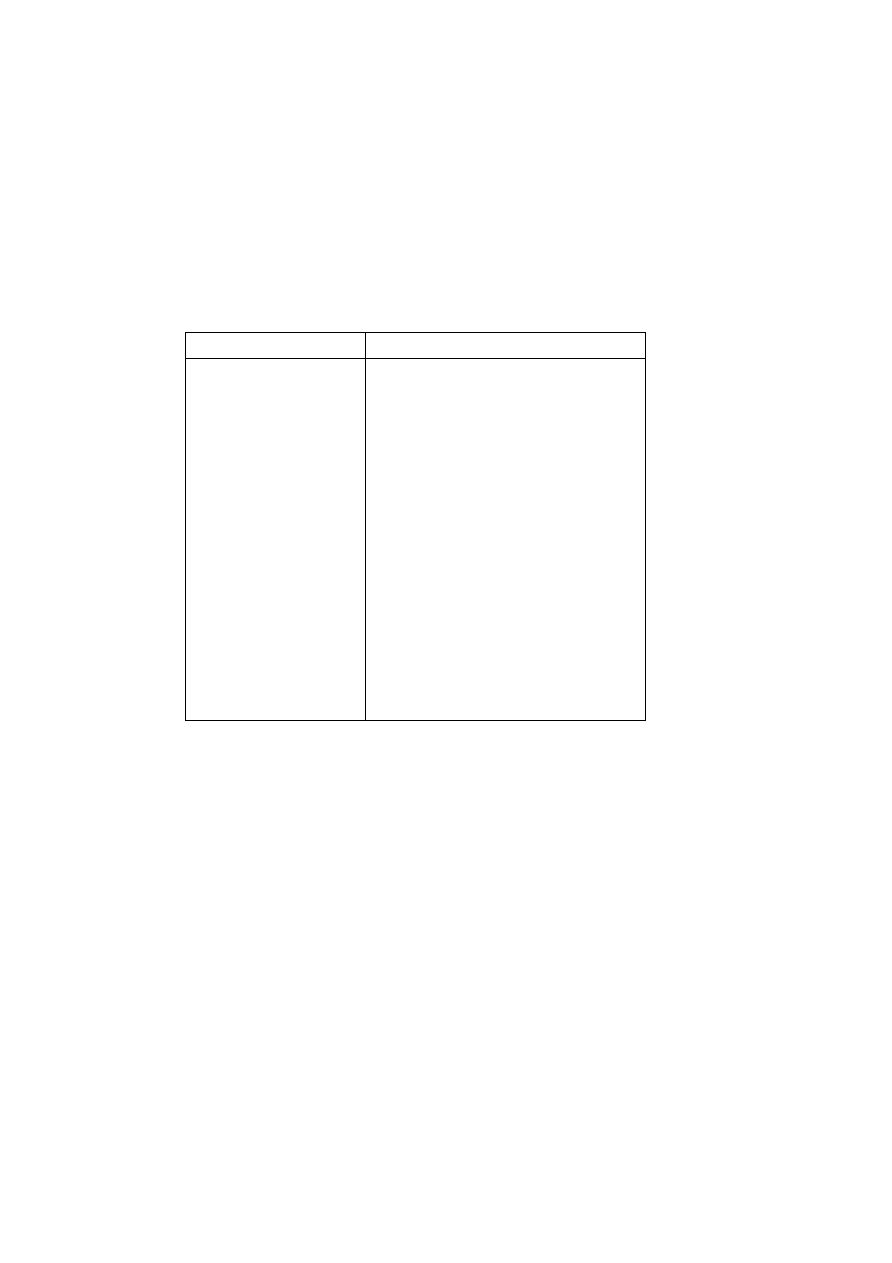ISO/IEC 10918-1 : 1993(E)
For simplicity of implementation, the divide by 2 in the prediction selections 5 and 6 of Table H.1 is done by an
arithmetic-right-shift of the integer values.
The difference between the prediction value and the input is calculated modulo 2
16
. In the decoder the difference is
16
, to the prediction.
H.1.2.2
Huffman coding of the modulo difference
The Huffman coding procedures defined in Annex F for coding the DC coefficients are used to code the modulo 2
16
differences. The table for DC coding contained in Tables F.1 and F.6 is extended by one additional entry. No extra bits
are appended after SSSS
=
16 is encoded. See Table H.2.
Table H.2 ­ Difference categories for lossless Huffman coding
SSSS
Difference values
1
0
0
1
1
­1,1
1
2
­3,­2,2,3
1
3
­7..­4,4..7
1
4
­15..­8,8..15
1
5
­31..­16,16..31
1
6
­63..­32,32..63
1
7
­127..­64,64..127
1
8
­255..­128,128..255
1
9
­511..­256,256..511
10
­1 023..­512,512..1 023
11
­2 047..­1 024,1 024..2 047
12
­4 095..­2 048,2 048..4 095
13
­8 191..­4 096,4 096..8 191
14
­16 383..­8 192,8 192..16 383
15
­32 767..­16 384,16 384..32 767
16
32 768
H.1.2.3
Arithmetic coding of the modulo difference
The statistical model defined for the DC coefficient arithmetic coding model (see F.1.4.4.1) is generalized to a two-
dimensional form in which differences coded for the sample to the left and for the line above are used for conditioning.
H.1.2.3.1
Two-dimensional statistical model
The binary decisions are conditioned on the differences coded for the neighbouring samples immediately above and
immediately to the left from the same component. As in the coding of the DC coefficients, the differences are classified
into 5 categories: zero(0), small positive (
+
S), small negative (­S), large positive (
+
L), and large negative (­L). The two
independent difference categories combine to give 25 different conditioning states. Figure H.2 shows the two-dimensional
array of conditioning indices. For each of the 25 conditioning states probability estimates for four binary decisions are
kept.
At the beginning of the scan and each restart interval the conditioning derived from the line above is set to zero for the
first line of each component. At the start of each line, the difference to the left is set to zero for the purposes of calculating
the conditioning.
134
CCITT Rec. T.81 (1992 E)